# EMT 282 Principles of Engineering Materials MECHANICAL PROPERTIES

• Slides: 47EMT 282 Principles of Engineering Materials MECHANICAL PROPERTIES OF METALS 6 -1 Dr. Nazuhusna Khalid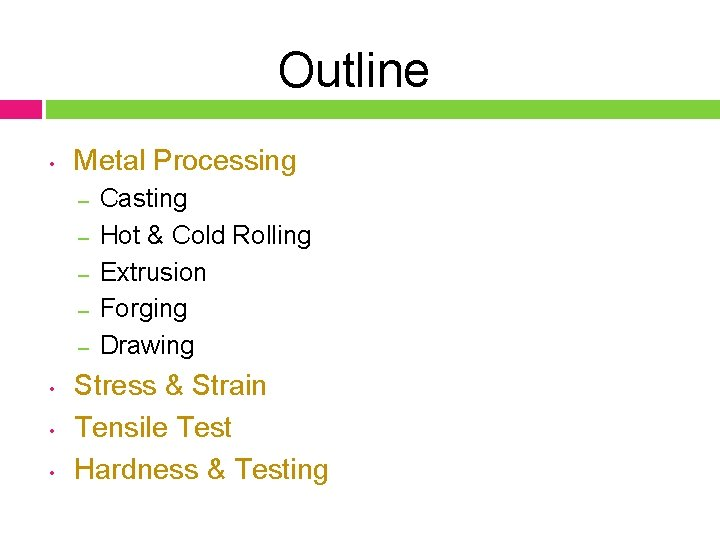Outline • Metal Processing – – – • • • Casting Hot & Cold Rolling Extrusion Forging Drawing Stress & Strain Tensile Test Hardness & TestingThe Processing of Metals and Alloys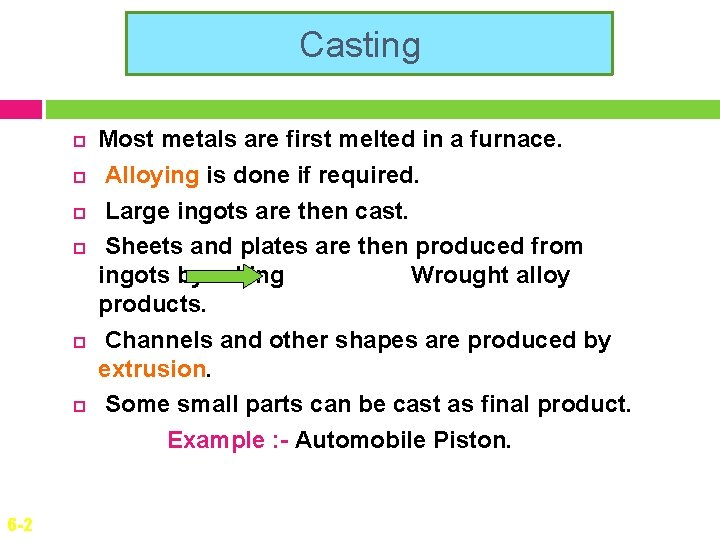Casting 6 -2 Most metals are first melted in a furnace. Alloying is done if required. Large ingots are then cast. Sheets and plates are then produced from ingots by rolling Wrought alloy products. Channels and other shapes are produced by extrusion. Some small parts can be cast as final product. Example : - Automobile Piston.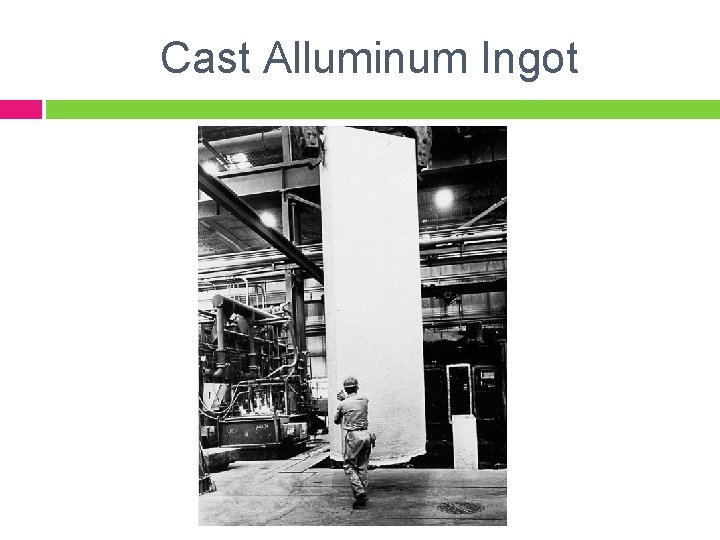Cast Alluminum Ingot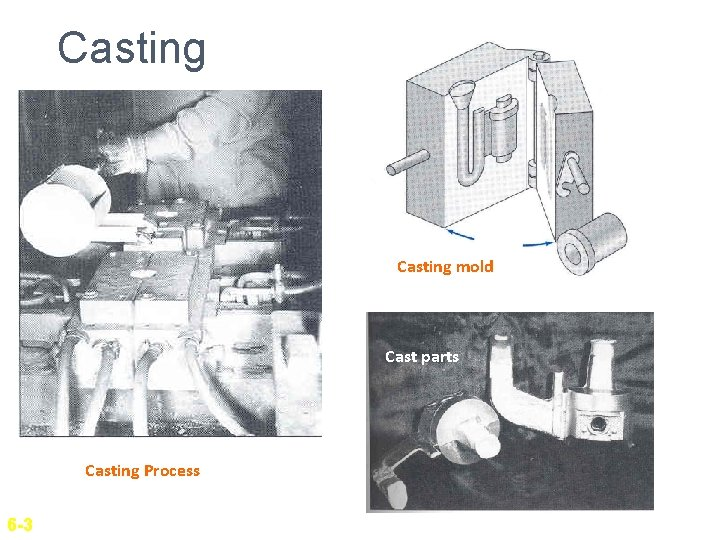Casting mold Cast parts Casting Process 6 -3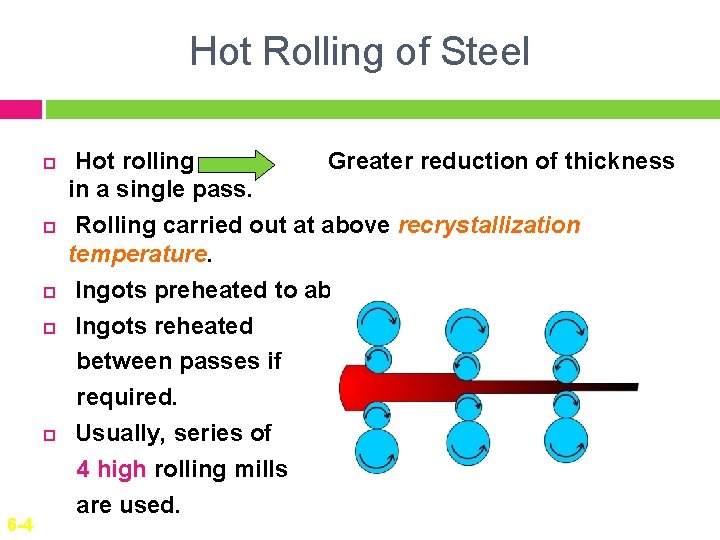Hot Rolling of Steel 6 -4 Hot rolling Greater reduction of thickness in a single pass. Rolling carried out at above recrystallization temperature. Ingots preheated to about 12000 C. Ingots reheated between passes if required. Usually, series of 4 high rolling mills are used.Cold Rolling of Metal Sheet 6 -5 Cold rolling is rolling performed below recrystallization temperature. This results in strain hardening. Hot rolled slabs have to be annealed before cold rolling. Series of 4 high rolling mills are usually used. Less reduction of thickness. Needs high power.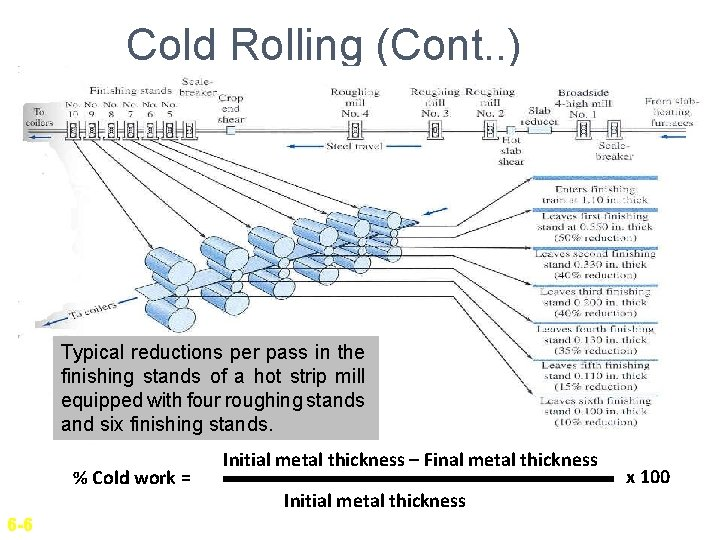Cold Rolling (Cont. . ) Typical reductions per pass in the finishing stands of a hot strip mill equipped with four roughing stands and six finishing stands. % Cold work = 6 -6 Initial metal thickness – Final metal thickness Initial metal thickness x 100Example Calculate the percent cold reduction after cold rolling 0. 040 -in-thick aluminum sheet to 0. 025 in. Solution: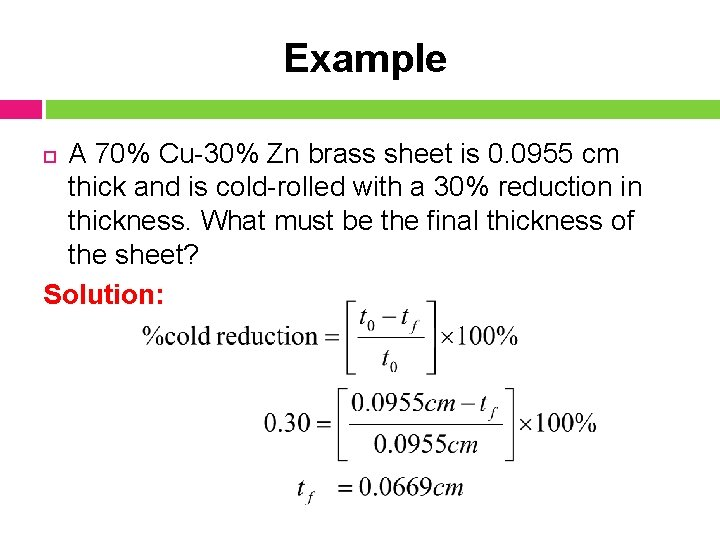Example A 70% Cu-30% Zn brass sheet is 0. 0955 cm thick and is cold-rolled with a 30% reduction in thickness. What must be the final thickness of the sheet? Solution: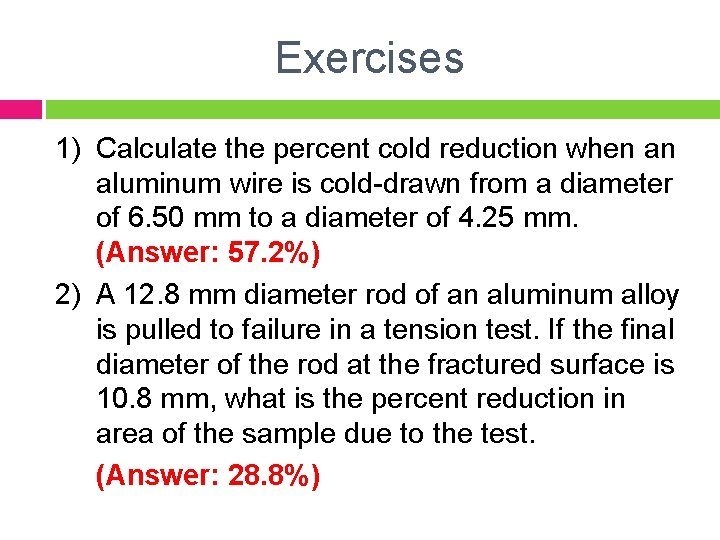Exercises 1) Calculate the percent cold reduction when an aluminum wire is cold-drawn from a diameter of 6. 50 mm to a diameter of 4. 25 mm. (Answer: 57. 2%) 2) A 12. 8 mm diameter rod of an aluminum alloy is pulled to failure in a tension test. If the final diameter of the rod at the fractured surface is 10. 8 mm, what is the percent reduction in area of the sample due to the test. (Answer: 28. 8%)Extrusion Die Extrusion- plastic-forming process in which a material under high pressure is reduced in cross section by forcing it through an opening in a die Metal under high pressure is forced through opening in a die. Common Products are cylindrical bar, hollow tubes from copper, aluminum etc. Normally done at high temperature. Indirect extrusion needs less power however there is limit on load applied. 6 -7 Container Metal Direct Extrusion Container Metal indirect Extrusion Figure 5. 9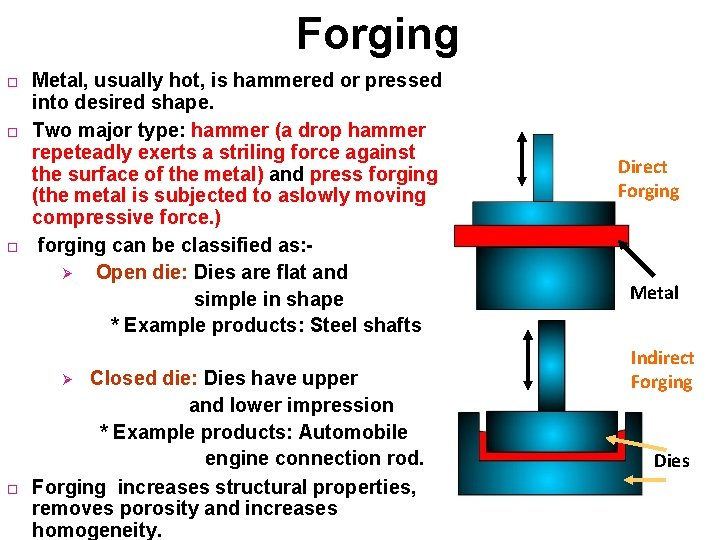Forging Metal, usually hot, is hammered or pressed into desired shape. Two major type: hammer (a drop hammer repeteadly exerts a striling force against the surface of the metal) and press forging (the metal is subjected to aslowly moving compressive force. ) forging can be classified as: Ø Open die: Dies are flat and simple in shape * Example products: Steel shafts Closed die: Dies have upper and lower impression * Example products: Automobile engine connection rod. Forging increases structural properties, removes porosity and increases homogeneity. Ø Direct Forging Metal Indirect Forging DiesDrawing Wire drawing : - Starting rod or wire is drawn through several drawing dies to reduce diameter. % cold work = Change in cross-sectional area Original area X 100 Wire or rod Carbide nib Deep drawing: - Used to shape cup like articles from flats and sheets of metals 6 -9Mechanical Properties of MetalsMechanical Properties of Metals Mechanical Properties refers to the behavior of material when external forces are applied Stress and strain ⇒ fracture For engineering point of view: allows to predict the ability of a component or a structure to withstand the forces applied to it For science point of view: what makes materials strong → helps us to design a better new one Learn basic concepts for metals, which have the simplest behavior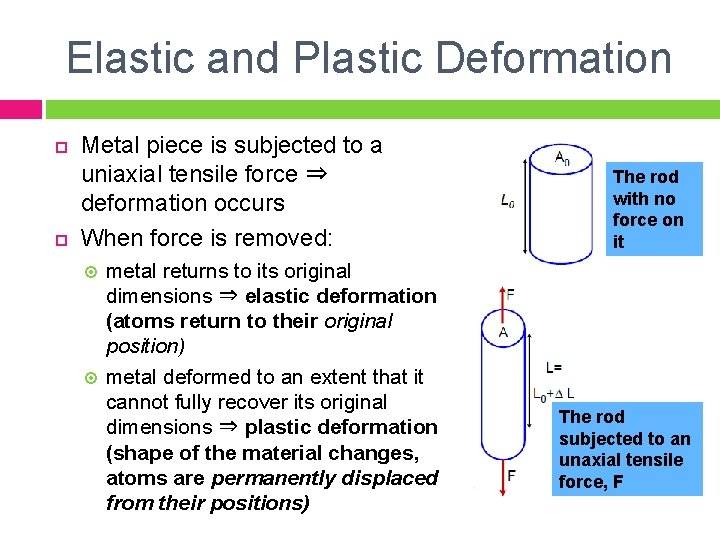Elastic and Plastic Deformation Metal piece is subjected to a uniaxial tensile force ⇒ deformation occurs When force is removed: metal returns to its original dimensions ⇒ elastic deformation (atoms return to their original position) metal deformed to an extent that it cannot fully recover its original dimensions ⇒ plastic deformation (shape of the material changes, atoms are permanently displaced from their positions) The rod with no force on it The rod subjected to an unaxial tensile force, F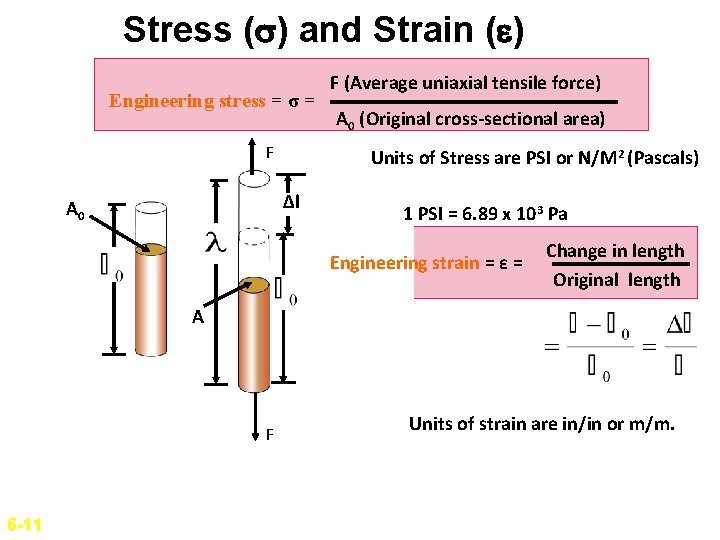Stress ( ) and Strain ( ) Engineering stress = σ = Engineering stress σF= Δl A 0 F (Average uniaxial tensile force) A 0 (Original cross-sectional area) Units of Stress are PSI or N/M 2 (Pascals) 1 PSI = 6. 89 x 103 Pa Engineering strain = ε = Change in length Original length A F 6 -11 Units of strain are in/in or m/m.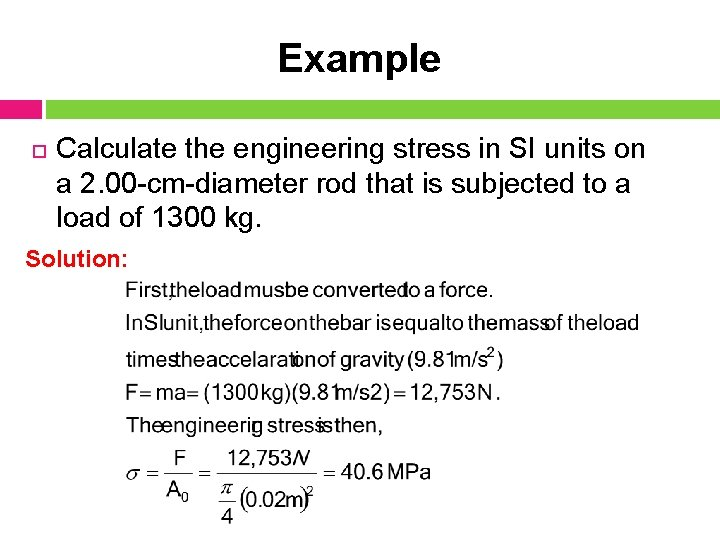Example Calculate the engineering stress in SI units on a 2. 00 -cm-diameter rod that is subjected to a load of 1300 kg. Solution: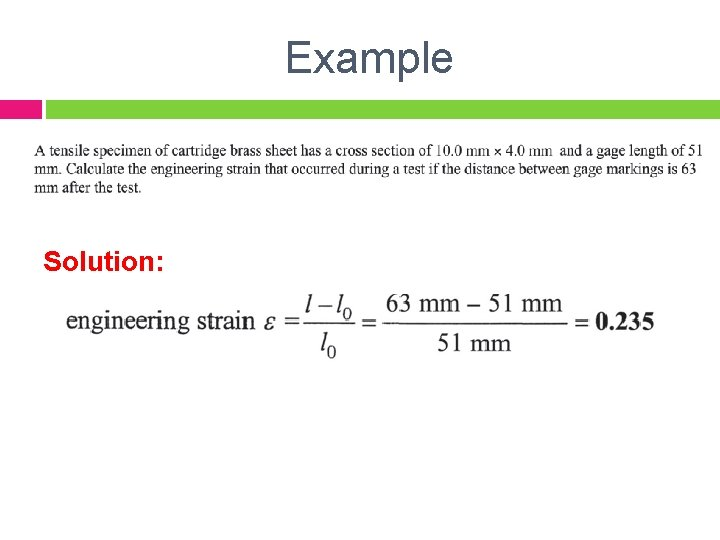Example Solution: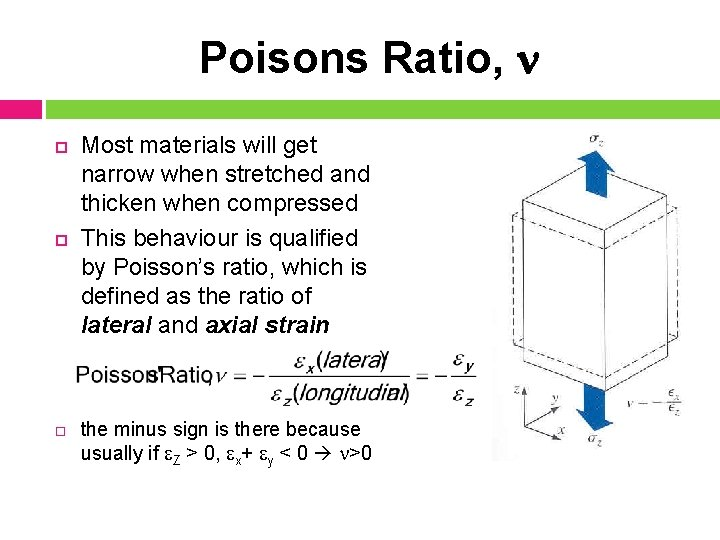Poisons Ratio, Most materials will get narrow when stretched and thicken when compressed This behaviour is qualified by Poisson’s ratio, which is defined as the ratio of lateral and axial strain the minus sign is there because usually if Z > 0, x+ y < 0 >0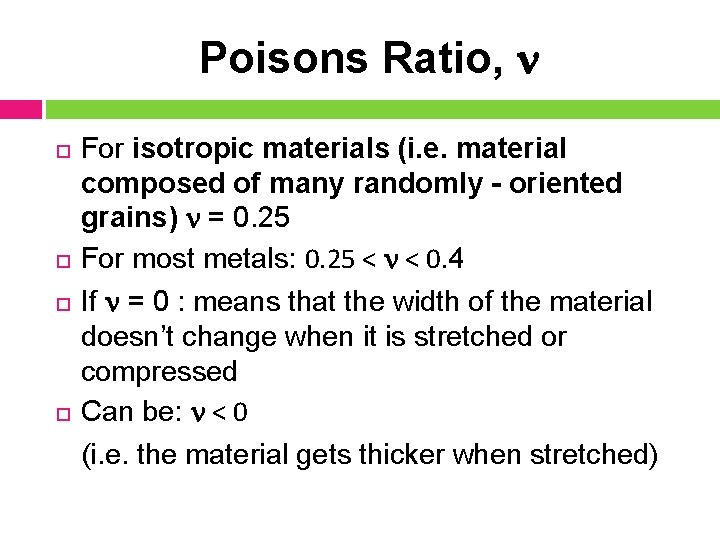Poisons Ratio, For isotropic materials (i. e. material composed of many randomly - oriented grains) = 0. 25 For most metals: 0. 25 < < 0. 4 If = 0 : means that the width of the material doesn’t change when it is stretched or compressed Can be: < 0 (i. e. the material gets thicker when stretched)Poisons Ratio Poisons ratio =. w 0 w Usually poisons ratio ranges from 0. 25 to 0. 4 for real materials. Example: Stainless steel 0. 28 Copper 0. 33 6 -12Shear Stress and Shear Strain 6 -13 If the shear force S acts over an area A, the shear stress : The shear strain is defined in terms of the amount of the shear displacement a divided by distance over which the shear acts:Mechanical Properties Obtained from Tensile Test Modulus of elasticity, E Yield strength at 0. 2 % offset Ultimate Tensile Strength (UTS) Percent elongation at fracture Percent reduction in area at fracture DuctilityModulus of elasticity, or Young’s Modulus Stress and strain are properties that don’t depend on the dimensions of the material (for small ε), just type of the material E– Young’s Modulus, Pa Comes from the linear range in the stress-strain diagram Behavior is related to atomic bonding between the atoms in a metal or alloy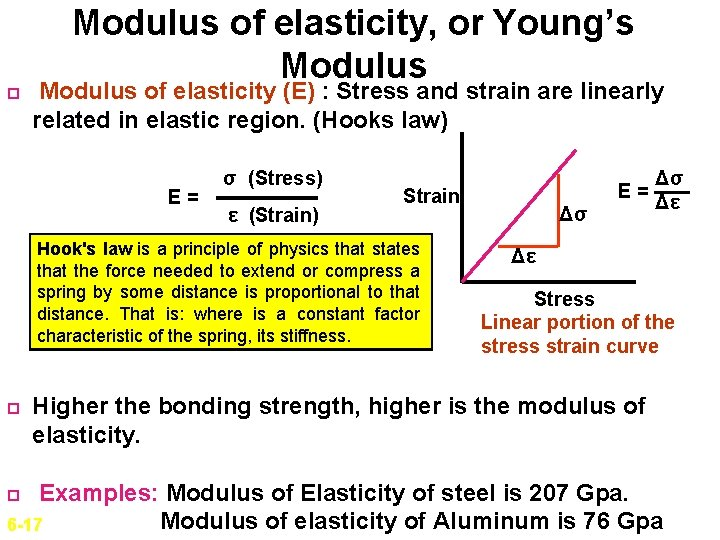Modulus of elasticity, or Young’s Modulus of elasticity (E) : Stress and strain are linearly related in elastic region. (Hooks law) E= σ (Stress) ε (Strain) Strain Hook's law is a principle of physics that states that the force needed to extend or compress a spring by some distance is proportional to that distance. That is: where is a constant factor characteristic of the spring, its stiffness. E= Δσ Δσ Δε Δε Stress Linear portion of the stress strain curve Higher the bonding strength, higher is the modulus of elasticity. Examples: Modulus of Elasticity of steel is 207 Gpa. Modulus of elasticity of Aluminum is 76 Gpa 6 -17Tensile test (to evaluate the strength of metal and alloys) Strength of materials can be tested by pulling the metal to failure. Load Cell Specimen Extensometer Force data is obtained from Load cell Strain data is obtained from Extensometer. 6 -14Tensile Test (Cont) Commonly used tension Test specimen Typical Stress-strain curve 6 -15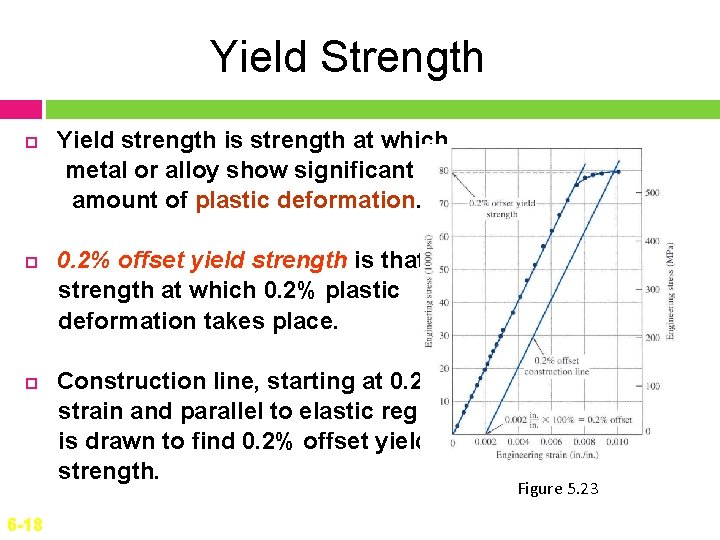Yield Strength 6 -18 Yield strength is strength at which metal or alloy show significant amount of plastic deformation. 0. 2% offset yield strength is that strength at which 0. 2% plastic deformation takes place. Construction line, starting at 0. 2% strain and parallel to elastic region is drawn to find 0. 2% offset yield strength. Figure 5. 23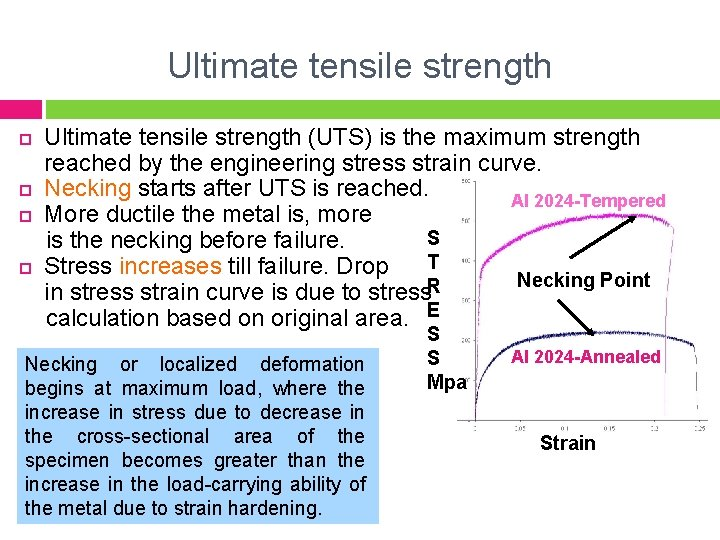Ultimate tensile strength (UTS) is the maximum strength reached by the engineering stress strain curve. Necking starts after UTS is reached. Al 2024 -Tempered More ductile the metal is, more S is the necking before failure. T Stress increases till failure. Drop Necking Point R in stress strain curve is due to stress calculation based on original area. E Necking or localized deformation begins at maximum load, where the increase in stress due to decrease in the cross-sectional area of the specimen becomes greater than the increase in the load-carrying ability of the metal due to strain hardening. S S Mpa Al 2024 -Annealed StrainTensile Strength Figure 7. 11 Typical engineering stress-strain behavior to fracture, point F. The tensile strength is indicated at point M. ¢ 35 Very familiar property and widely used for identification of a material. It is used for the purposes of specifications and for quality control of a product.Ductility ¢ ¢ 36 It is a measure of the degree of plastic deformation that has been sustained at fracture. A material that experiences very little or no plastic deformation upon fracture is termed brittle. Ductile material – Significant plastic deformation and energy absorption (toughness) before fracture. Characteristic feature of ductile material – necking Brittle material – Little plastic deformation or energy absorption before fracture. Characteristic feature of brittle materials – fracture surface perpendicular to the stress. Schematic representations of tensile stress-strain behavior for brittle and ductile materials loaded to fracture.Percent Elongation Percent elongation is a measure of ductility of a material. It is the elongation of the metal before fracture expressed as percentage of original length. Final length – initial Length % Elongation = Initial Length Measured using a caliper fitting the fractured metal together. Example: - Percent elongation of pure aluminum is 35% For 7076 -T 6 aluminum alloy it is 11%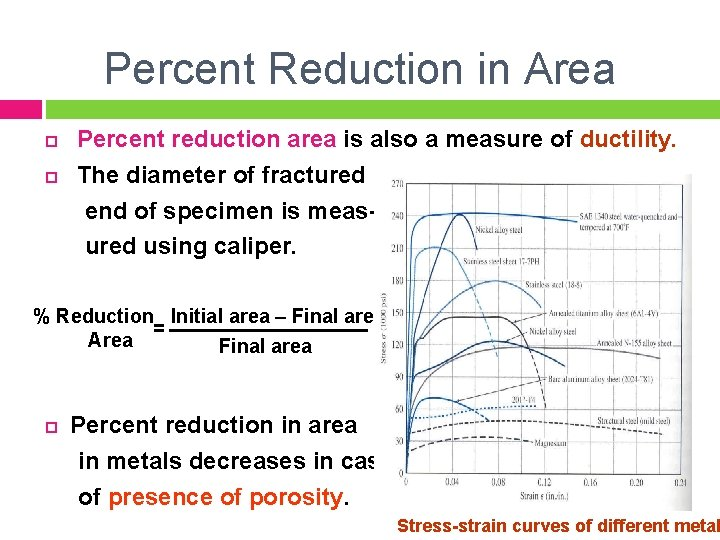Percent Reduction in Area Percent reduction area is also a measure of ductility. The diameter of fractured end of specimen is measured using caliper. % Reduction Initial area – Final area = Area Final area Percent reduction in area in metals decreases in case of presence of porosity. Stress-strain curves of different metal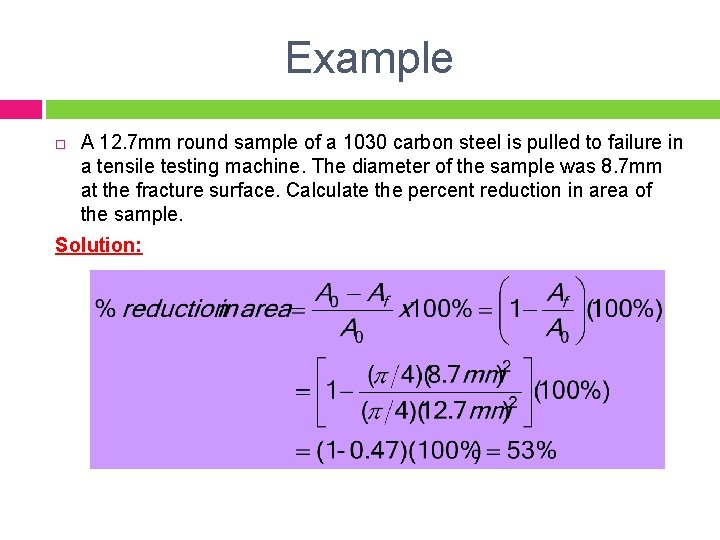Example A 12. 7 mm round sample of a 1030 carbon steel is pulled to failure in a tensile testing machine. The diameter of the sample was 8. 7 mm at the fracture surface. Calculate the percent reduction in area of the sample. Solution:True Stress – True Strain True stress and true strain are based upon instantaneous crosssectional area and length. True stress is the stress determined by the instantaneous load acting on the instantaneous crosssectional area True strain is the rate of instantaneous increase in the instantaneous gauge lengthTrue Stress – True Strain 41 True stress and true strain are based upon instantaneous cross-sectional area and length. F True Stress = σt = True Strain = εt = True stress is always greater than engineering stress. Ai (instantaneous area)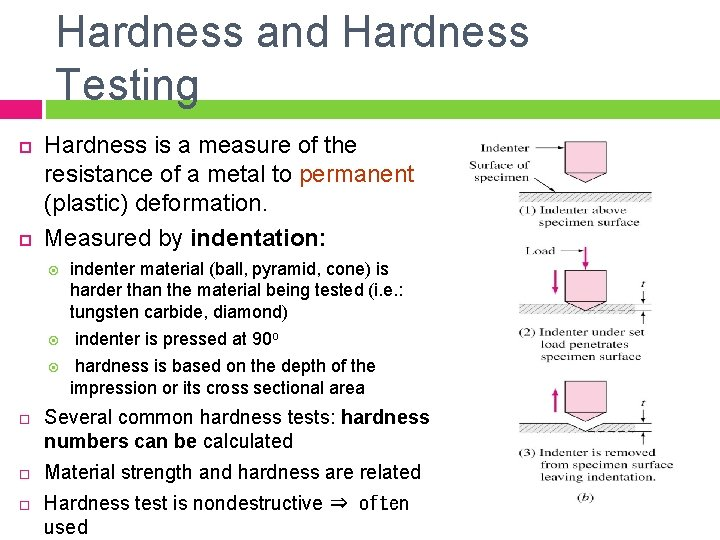Hardness and Hardness Testing Hardness is a measure of the resistance of a metal to permanent (plastic) deformation. Measured by indentation: indenter material (ball, pyramid, cone) is harder than the material being tested (i. e. : tungsten carbide, diamond) indenter is pressed at 90 o hardness is based on the depth of the impression or its cross sectional area Several common hardness tests: hardness numbers can be calculated Material strength and hardness are related Hardness test is nondestructive ⇒ often usedHardness Tests 43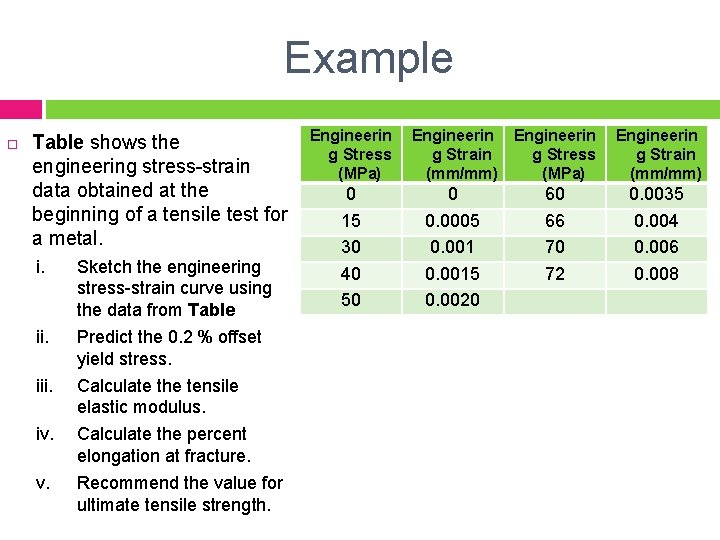Example Table shows the engineering stress-strain data obtained at the beginning of a tensile test for a metal. i. Sketch the engineering stress-strain curve using the data from Table ii. Predict the 0. 2 % offset yield stress. iii. Calculate the tensile elastic modulus. iv. Calculate the percent elongation at fracture. v. Recommend the value for ultimate tensile strength. Engineerin g Stress (MPa) 0 15 30 40 50 Engineerin g Strain g Stress (mm/mm) (MPa) 0 0. 0005 0. 0015 0. 0020 60 66 70 72 Engineerin g Strain (mm/mm) 0. 0035 0. 004 0. 006 0. 008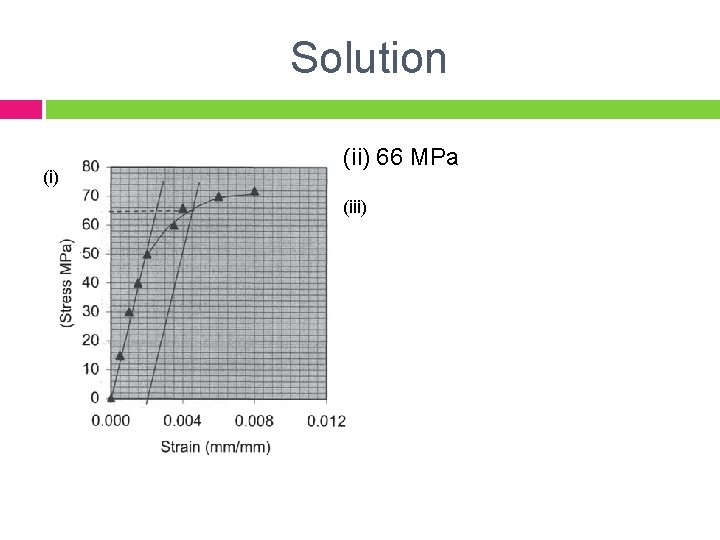Solution (i) (ii) 66 MPa (iii)Example A tensile specimen of aluminum alloy is tested to fracture. At the fracture point, it has an engineering stress 180 MPa and engineering strain 34%. Calculate (a) the true stress at fracture and (b) the true strain at fracture. SolutionSummary Stress and strain: These are size‐independent measures of load and displacement, respectively. Elastic behavior: This reversible behavior often shows a linear relation between stress and strain. To minimize deformation, select a material with a large elastic modulus (E or G). Plastic behavior: This permanent deformation behavior occurs when the tensile (or compressive) uniaxial stress reaches y. Toughness: The energy needed to break a unit volume of material. Ductility: The plastic strain at failure.